EViews provides you with the ability to examine and work with the gradients of the objective function for a variety of estimation objects. Examining these gradients can provide useful information for evaluating the behavior of your nonlinear estimation routine, or can be used as the basis of various tests of specification.
Since EViews provides a variety of estimation methods and techniques, the notion of a gradient is a bit difficult to describe in casual terms. EViews will generally report the values of the first-order conditions used in estimation. To take the simplest example, ordinary least squares minimizes the sum-of-squared residuals: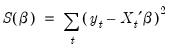(59.24)
The first-order conditions for this objective function are obtained by differentiating with respect to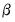, yielding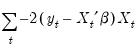(59.25)
EViews allows you to examine both the sum and the corresponding average, as well as the value for each of the individual observations. Furthermore, you can save the individual values in series for subsequent analysis.
The individual gradient computations are summarized in the following table:

 Least squares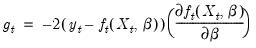Weighted least squares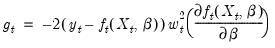Two-stage least squares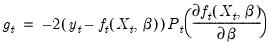Weighted two-stage least squares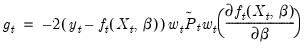Maximum likelihood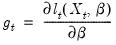where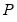and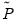are the projection matrices corresponding to the expressions for the estimators in “Instrumental Variables and GMM”, and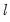is the log likelihood contribution function.
Note that the expressions for the regression gradients are adjusted accordingly in the presence of ARMA error terms.
To view the summary of the gradients, select View/Gradients and Derivatives/Gradient Summary, or View/Gradients/Summary. EViews will display a summary table showing the sum, mean, and Newton direction associated with the gradients. Here is an example table from a nonlinear least squares estimation equation: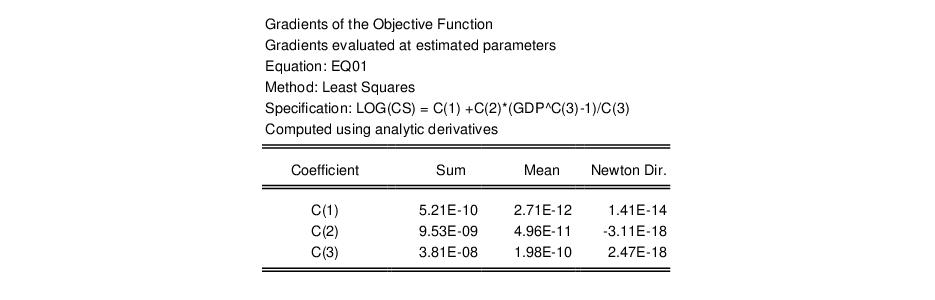There are several things to note about this table. The first line of the table indicates that the gradients have been computed at estimated parameters. If you ask for a gradient view for an estimation object that has not been successfully estimated, EViews will compute the gradients at the current parameter values and will note this in the table. This behavior allows you to diagnose unsuccessful estimation problems using the gradient values.
Second, you will note that EViews informs you that the gradients were computed using analytic derivatives. EViews will also inform you if the specification is linear, if the derivatives were computed numerically, or if EViews used a mixture of analytic and numeric techniques. We remind you that all MA coefficient derivatives are computed numerically.
Lastly, there is a table showing the sum and mean of the gradients as well as a column labeled “Newton Dir.”. The column reports the non-Marquardt adjusted Newton direction used in first-derivative iterative estimation procedures (see “First Derivative Methods”).
In the example above, all of the values are “close” to zero. While one might expect these values always to be close to zero when evaluated at the estimated parameters, there are a number of reasons why this will not always be the case. First, note that the sum and mean values are highly scale variant so that changes in the scale of the dependent and independent variables may lead to marked changes in these values. Second, you should bear in mind that while the Newton direction is related to the terms used in the optimization procedures, EViews’ test for convergence does not directly use the Newton direction. Third, some of the iteration options for system estimation do not iterate coefficients or weights fully to convergence. Lastly, you should note that the values of these gradients are sensitive to the accuracy of any numeric differentiation.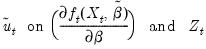(59.26)
where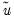are the estimated residuals under the restricted (null) model, and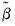are the estimated coefficients. The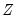are a set of “misspecification indicators” which correspond to departures from the null hypothesis.